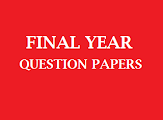# Dibrugarh University (M.Com - Distance) - Financial Management(Nov-Dec' 2015)2015
(December held in January 2016)
COMMERCE
Paper: 201
(Financial Management)
Full Marks: 80
Time: 3 hours
The figures in the margin indicate full marks for the questions

1. (a) Give the definition and scope of Financial Management. Narrate briefly the functions of Financial Management. 3+5+8=16
Or
(b) Discuss, in detail, the modern concept of finance. In what respects, the modern concept differs from the traditional concept?                             10+6=16

2. (a) Explain the different techniques of analysis of financial statements.                           16
Or
(b) Write brief notes on:                               4x4=16
1)      Acid Test ratio.
2)      Debt-Turnover ratio.
3)      Capital gearing ratio.
4)      DuPont System of Financial Analysis.

3. (a) Examine the merits and limitations of debentures as a source of long-term finance of companies.                 8+8=16
Or

(b) Write explanatory notes on:                                8+8=16
1)      Rights Issue of Equity Shares.
2)      Term Loan.
4. (a) Analyze the advantages and disadvantages of Trade Credit as a source of short-term financing.    8+8=16
Or
(b) Make a comparison between unsecured borrowing and secured borrowing as a source of short-term finance.     16
5. (a) Write a note on basic financial derivatives and the functions of Future markets.                                     8+8=16
Or
(b) Critically analyze the recent trends in global derivatives market.                                     16
***